# Top 10 Features of TI-84 Plus for Taking the ACT®

Posted 04/06/2018 by Tom Reardon, @tomreardon3

There are 60 questions on the math section of the ACT and you only have 60 minutes to complete them. The TI-84 Plus graphing calculator is allowed during the entire portion of the math section of the ACT.* The questions get more difficult as the problem number increases, so you should aim to do questions #1-20 in 30 seconds each, #20-40 in 60 seconds each, and #40- 60 in 90 seconds each, on average.  You can use your TI graphing calculator to work through problems more efficiently, help verify answers, and model a difficult word problem.  Below are 10 features on the TI-84 Plus that help students do their best work on the ACT.

1: Calculating Complex Numbers

Come across a problem that uses complex numbers? Fear not! You can change the TI-84 Plus into a + bi (complex) mode. Do this by pressing the mode key on your calculator and on the 7th row, you’ll see the a + bi (complex) mode. Once you switch your calculator to that mode, you can add, subtract, multiply, and divide complex numbers. You can even calculate the powers of i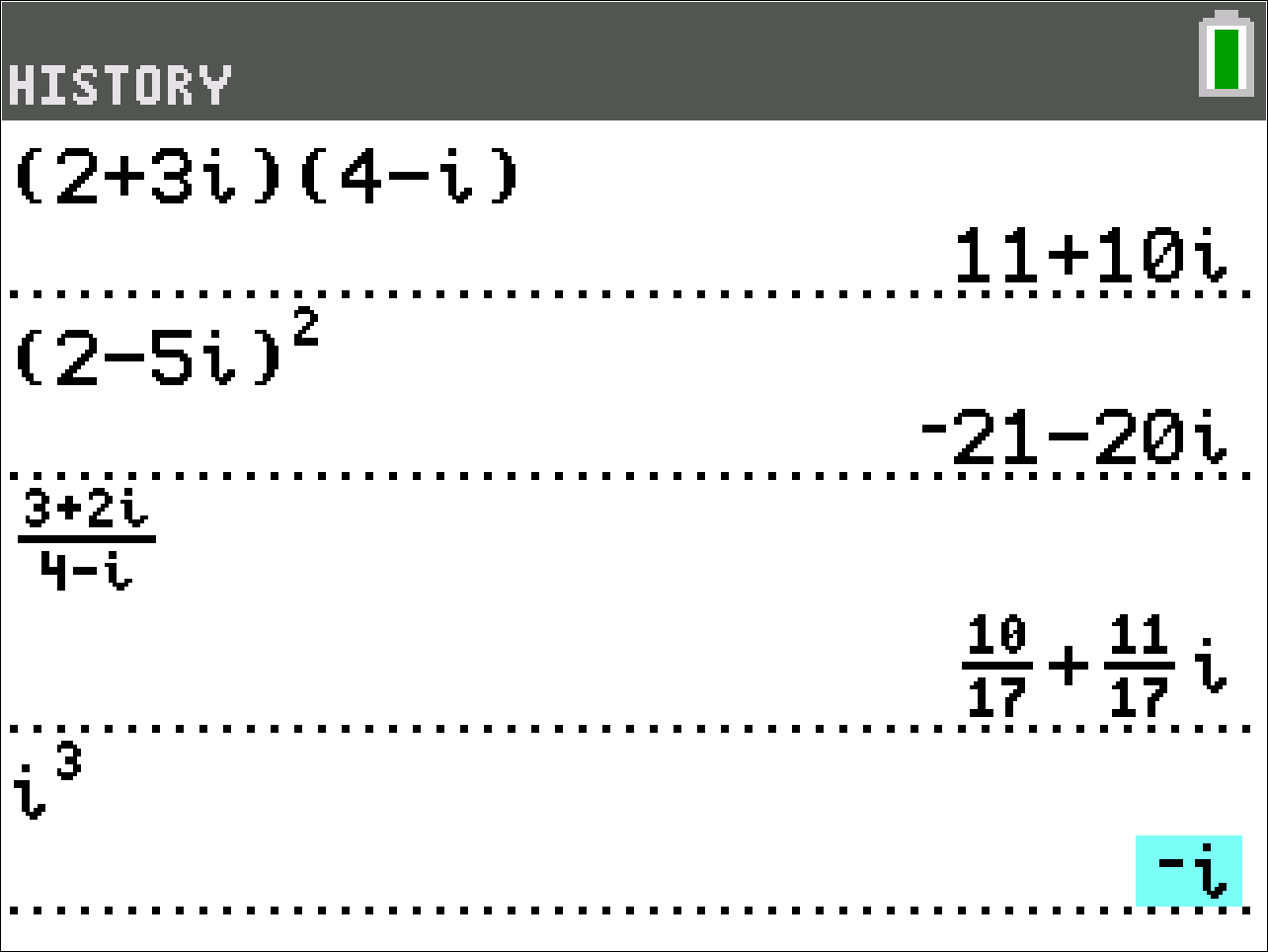2: Evaluating Functions

On the ACT, you are often asked to evaluate functions and composition of functions. You can save time by using a feature on the TI-84 Plus that you normally use to graph functions.  For example, you might see a problem like this:

If a(x) = 3x-2 and b(x) = x² +4 then b(a(4)) = ?

You can store the functions in Y₁ and Y₂ to help you answer this question.  Press the y= key, then in Y₁ enter in the first function, 3x-2.  In Y₂, enter in the second function, x² +4.  Then on the home screen, you can use that to do b(a(4)) in this case would be Y₂ (Y₁ (4)). You can find Y₁ and Y₂ by pressing the alpha key and then trace (f4).

Now that you know this trick, you can use it to do simple things like evaluating functions or take it to the next level and add, subtract, multiply and divide functions. You can even evaluate the composition of functions like in the example.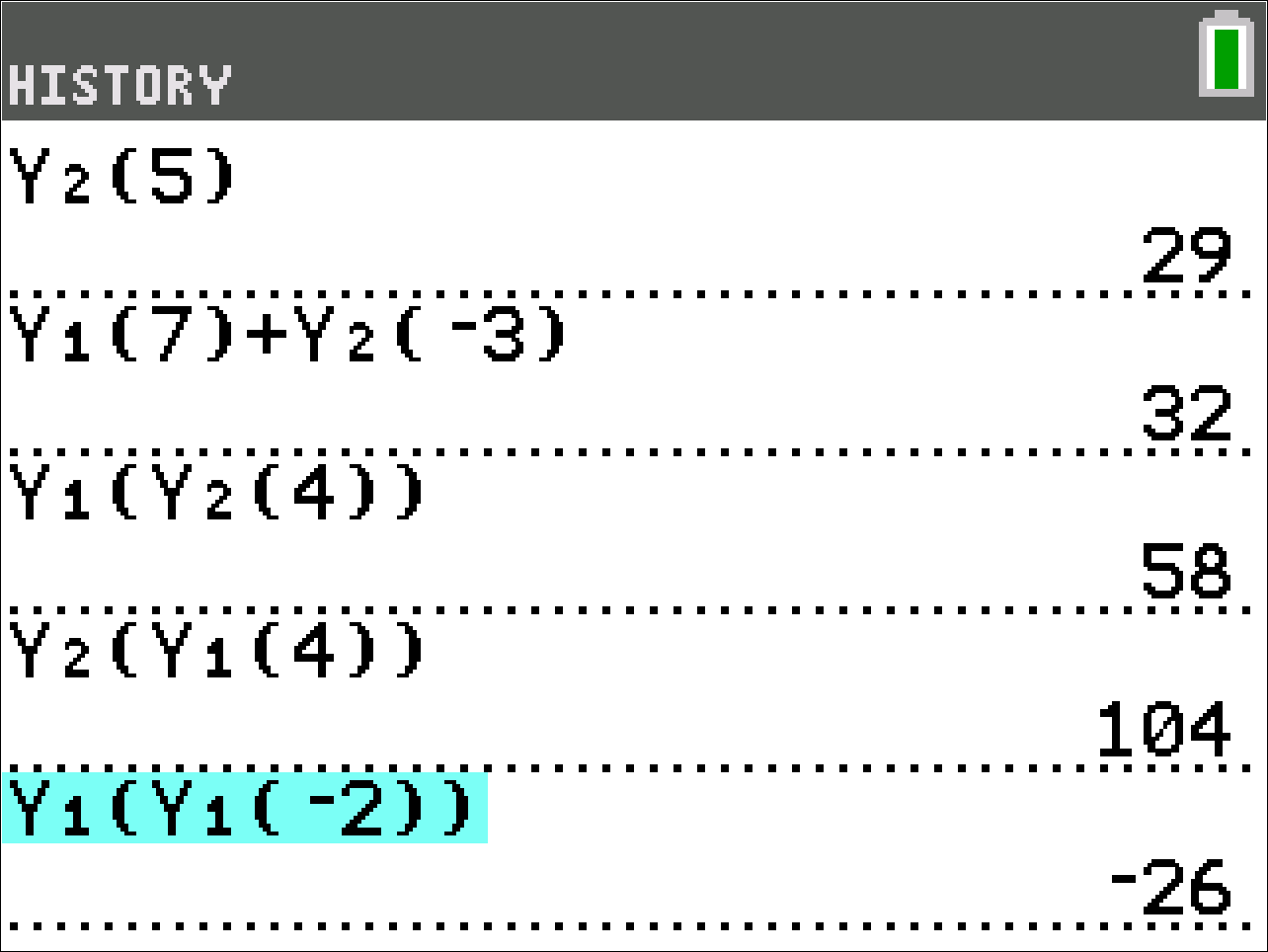3: Evaluate Expressions Involving More Than One Variable

Sometimes you will need to evaluate expressions on the ACT.  In the screenshot below, you can see an example on how to evaluate various expressions for A = – 4 and B = – 3.  This is possible by using the sto → key on the TI-84 Plus. You can use this feature to assign or store values to a variable.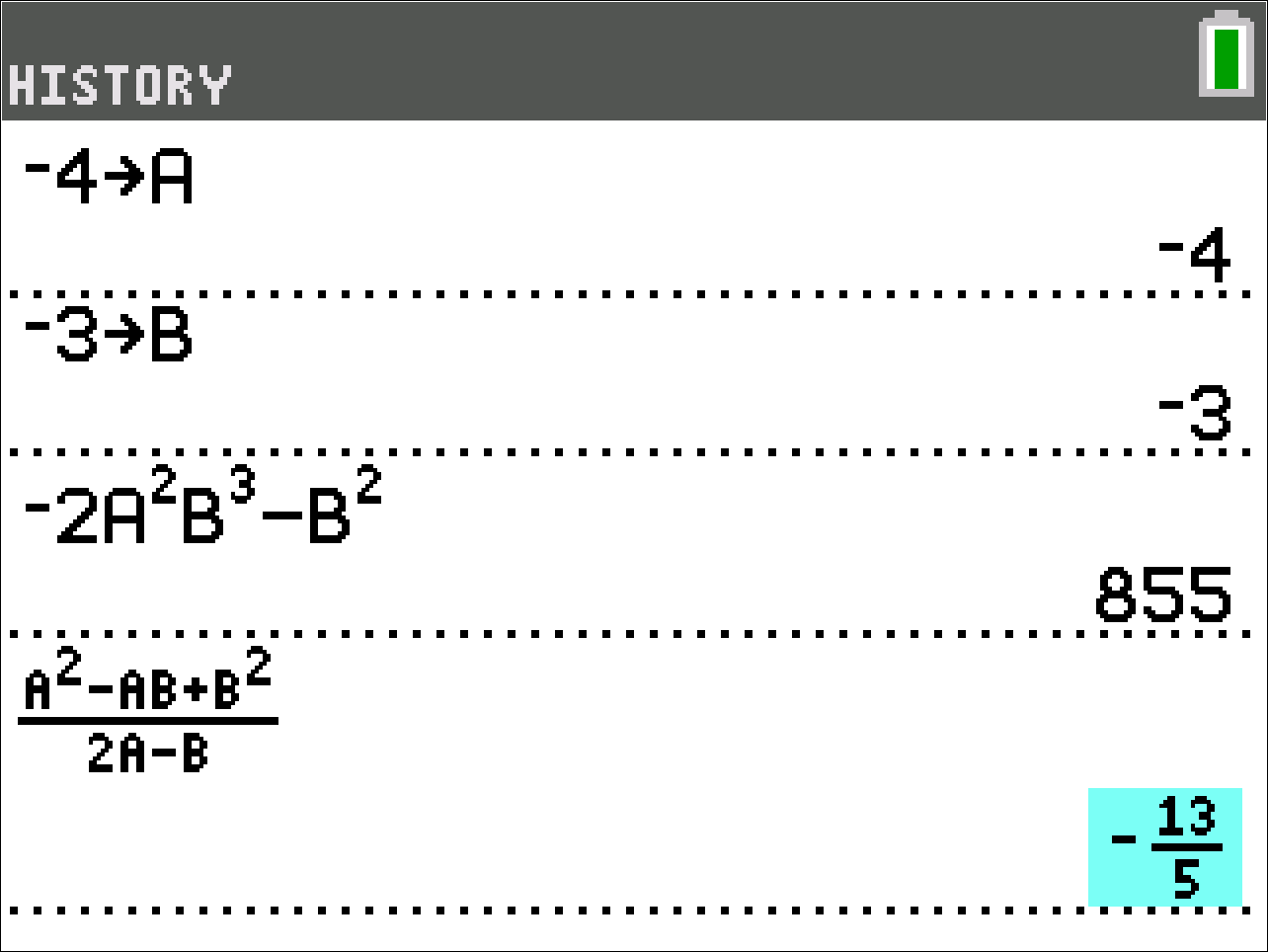4: Absolute Value – Discover Math Conceptually, Graphically, Visually

When you are faced with an absolute value equation or an inequality problem on the ACT, it might be helpful to see the graph before starting to solve the problem. Students sometimes start solving algebraically and might miss that there are two possible solutions.  For example, there might be a problem like this:

Solve: |2x-5|=7

In this problem, you can graph |2x-5| in Y₁ and 7 in Y₂.  Once you press the graph button, you’ll see that there are clearly two solutions. One solution is at  x = – 1 and the other at x = 6.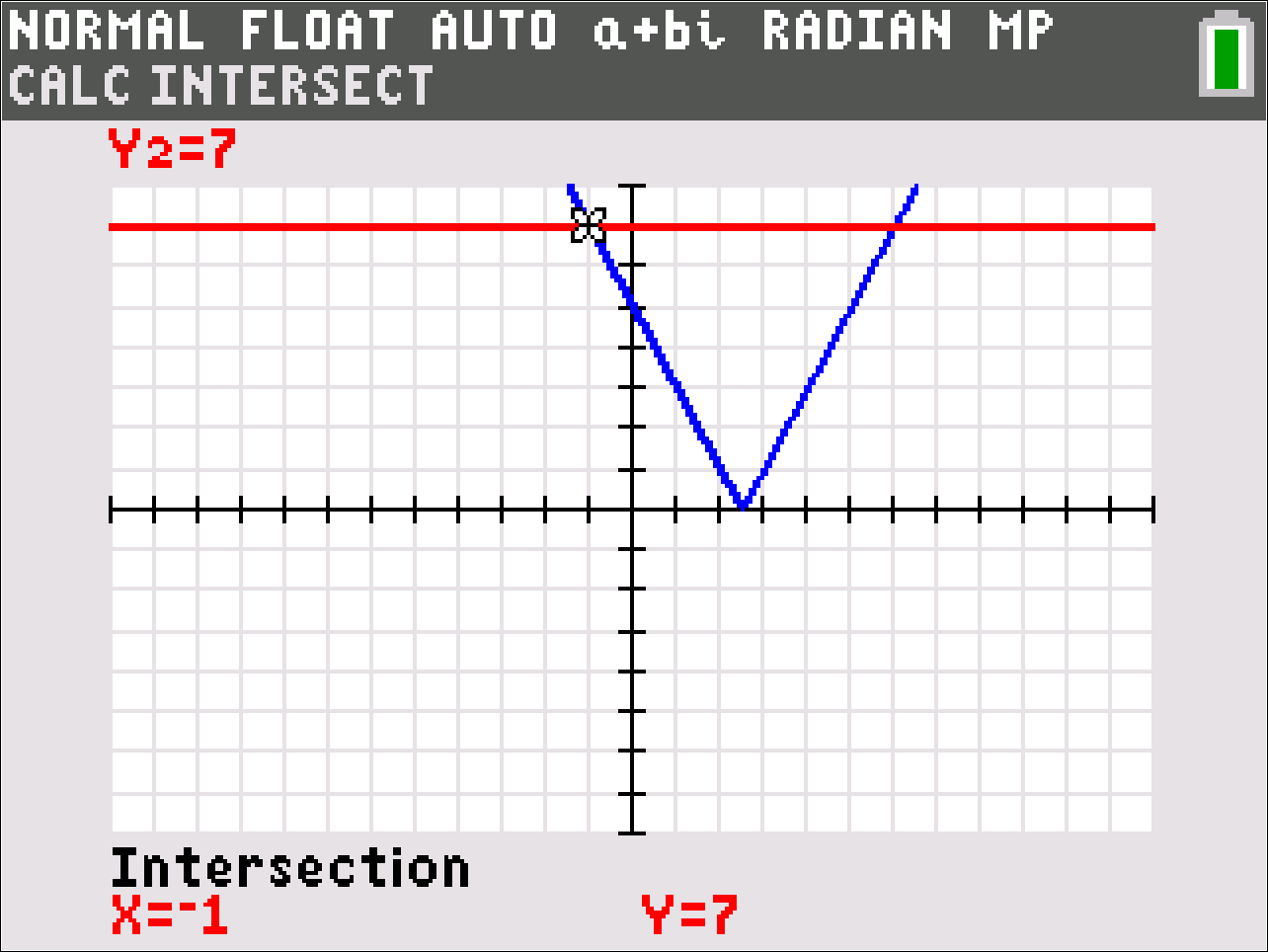5: Using Matrices

Working with matrices can be a tedious process. There are many chances to make careless mistakes by doing the wrong calculations or misplacing a minus sign. For this reason, I suggest that students save some time and do matrix multiplication, evaluating the determinant, and solving systems on the calculator.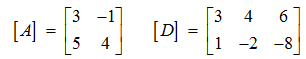Let’s say that you encounter a problem with the matrices above. You can enter the matrices in the TI-84 Plus by pressing the 2nd key then the x⁻¹ key then arrowing over to EDIT.  Once you enter the matrices, you can multiply two matrices together (shown in the screenshot below). You can find the determinant by pressing the 2nd key then the x⁻¹ button, then arrowing over to MATH.  To solve systems, you can use the rref (row reduced echelon form) command that is located toward the bottom of the menu.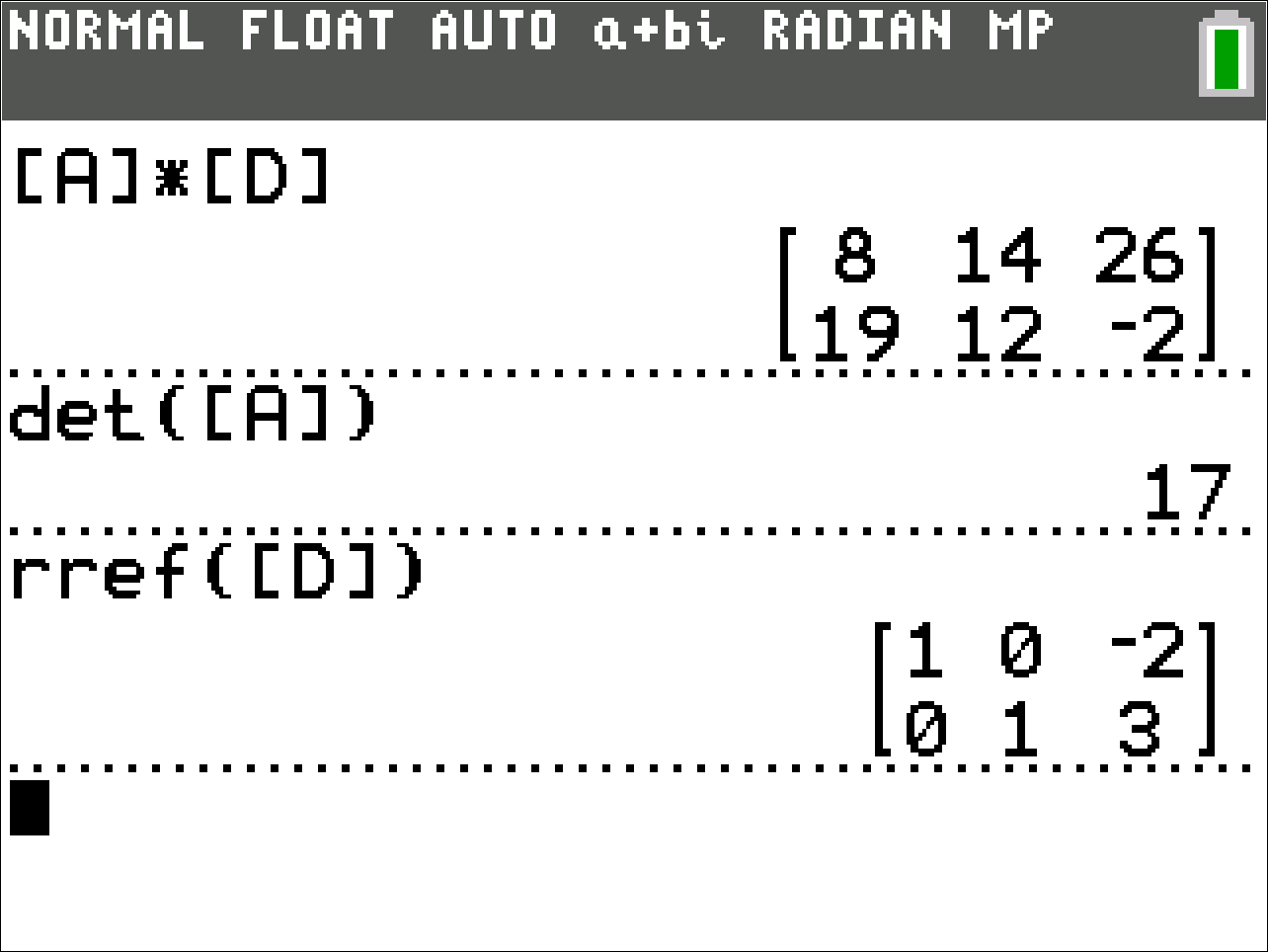6: Solving Trig Ratios and Identities

For trig questions, it is important to make sure your calculator is in DEGREE mode. You can check by pressing the mode key and going to down to the third row.

Two identities that are referenced often on the ACT are: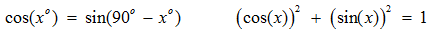These are true for all values of x.  You can refresh your memory by using the calculator like shown in the screenshot below.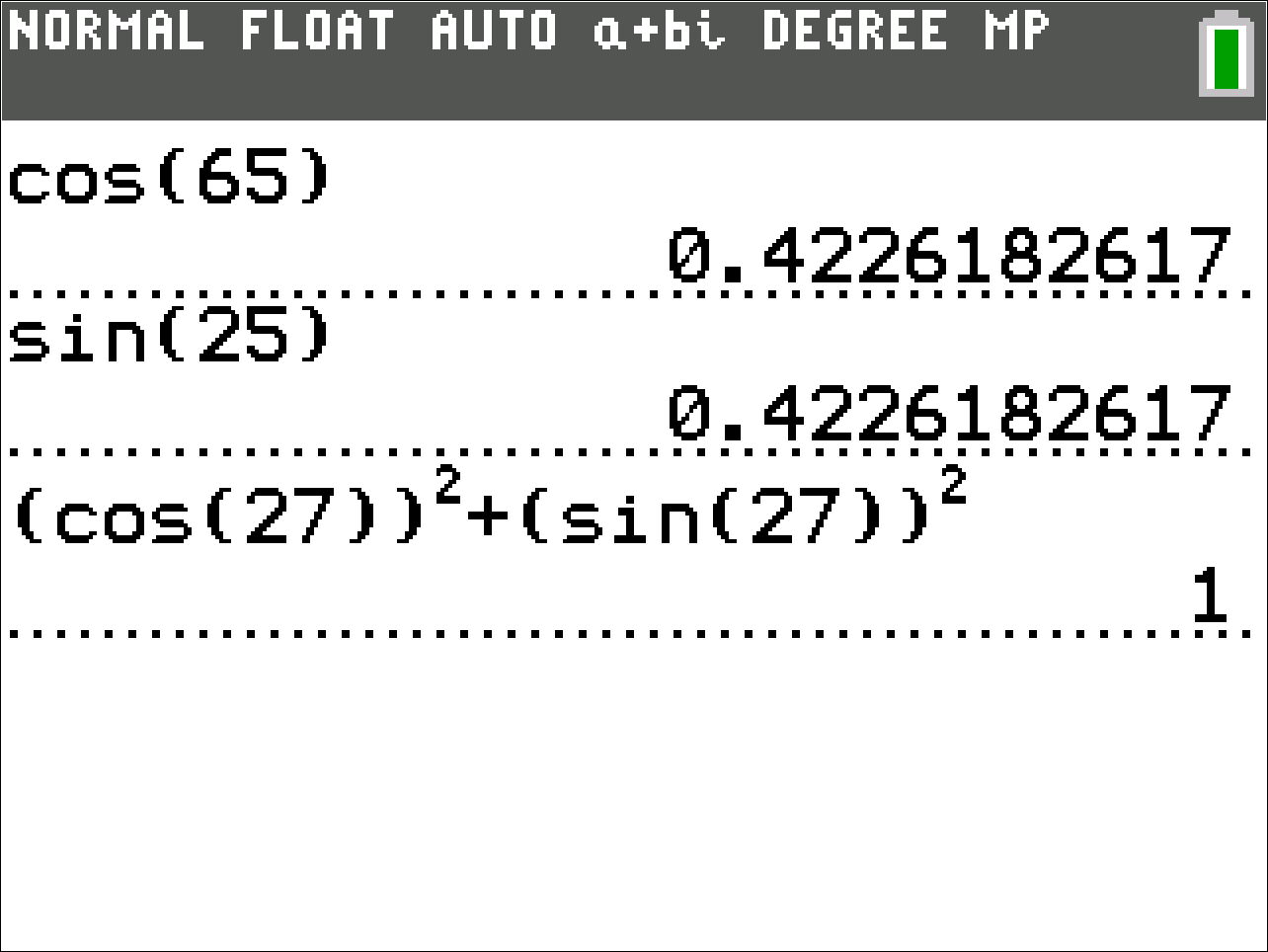7: Translate and Dilate Function

You will need to be able to graph functions that have been shifted up/ down, left/ right and dilated (transformations).
The Transformation app on the TI-84 Plus can help students practice those concepts. Turn on the transformation graphing feature on your calculator by pressing the app key and navigating to the Transformation App (Transfrm).  The animated gif below shows an example of how the Transformation app can help students understand vertical shifts, horizontal shifts, and dilations.

You can use up to four parameters: A, B, C, D to transform a function. Most functions are supported.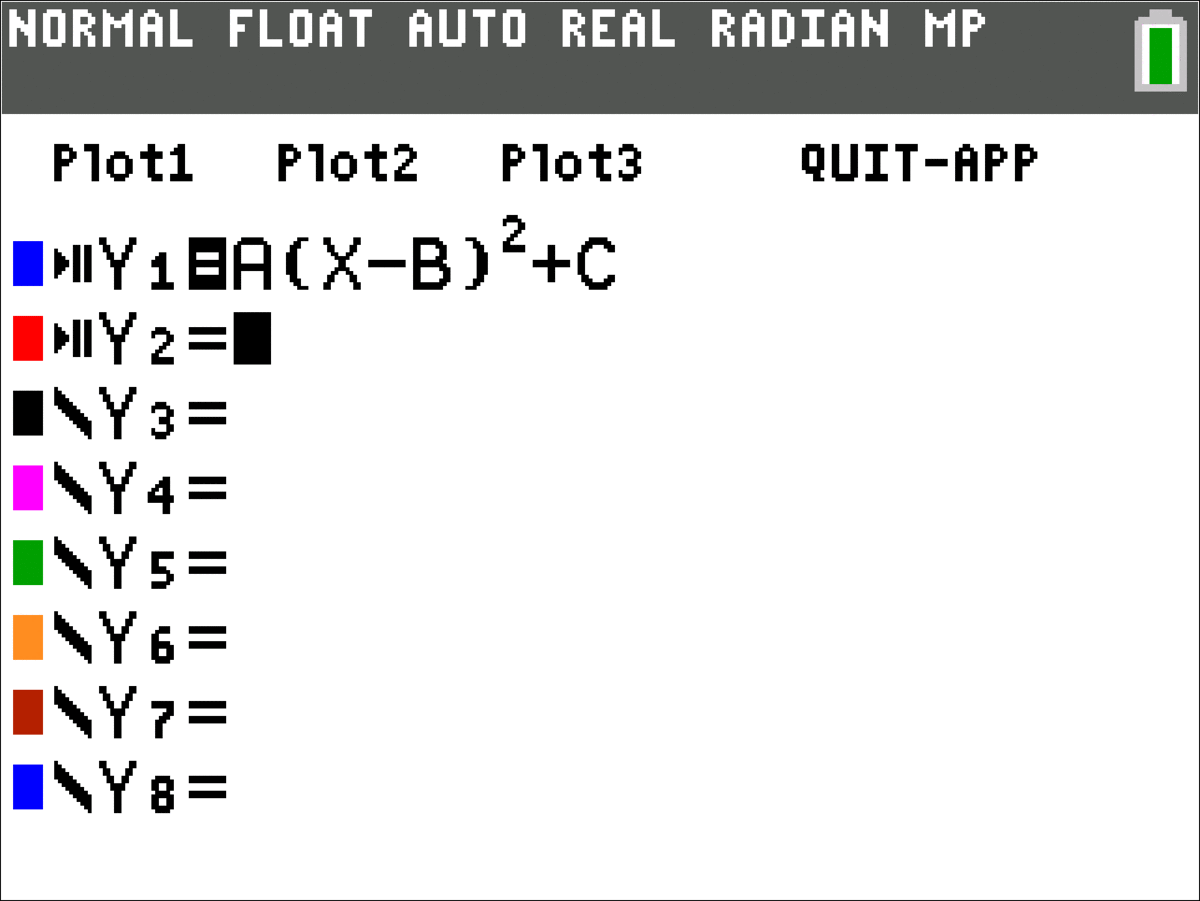8: Fractions

Sometimes, under pressure, even fractions can start to look intimidating.  If fractions are causing you some grief, then use your calculator to simplify, add, subtract, multiply, and divide fractions. You can also rewrite fractions as decimals.  Press the alpha key then the y= key to pull up the fraction menu.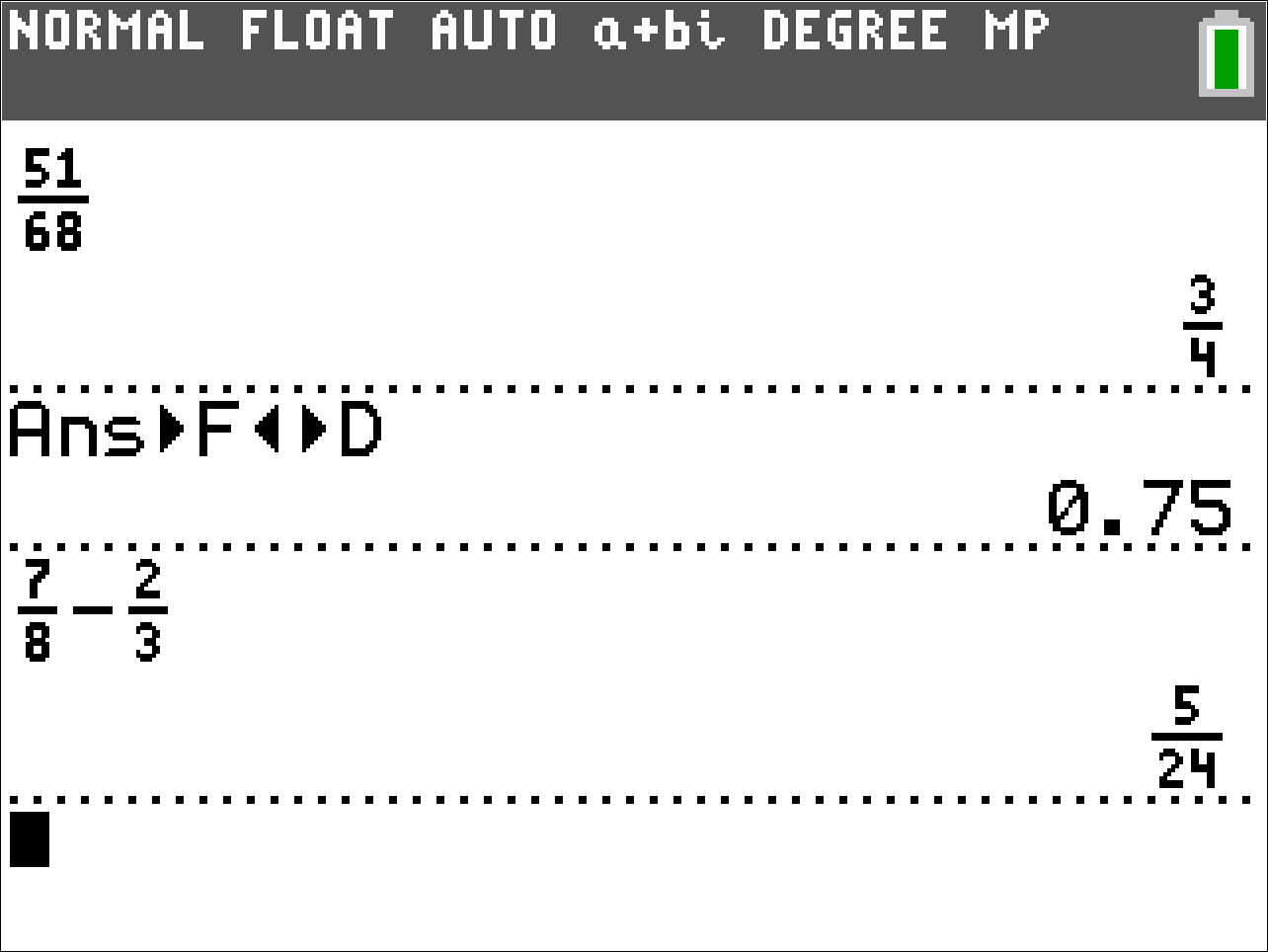9: Solve Systems of Equations Graphically

You will be asked to solve systems of equations on the ACT. After you solve the problem algebraically, I suggest you check your solution graphically, using your TI-84 Plus.  On the ACT, you might see a question like this:

Solve:
2x - 3y =  -4
x + y= -1

Before you can graph the functions, make sure to solve for y first.  After you graph your functions, press the 2nd key and then the trace key and then select intersect to find the intersection point.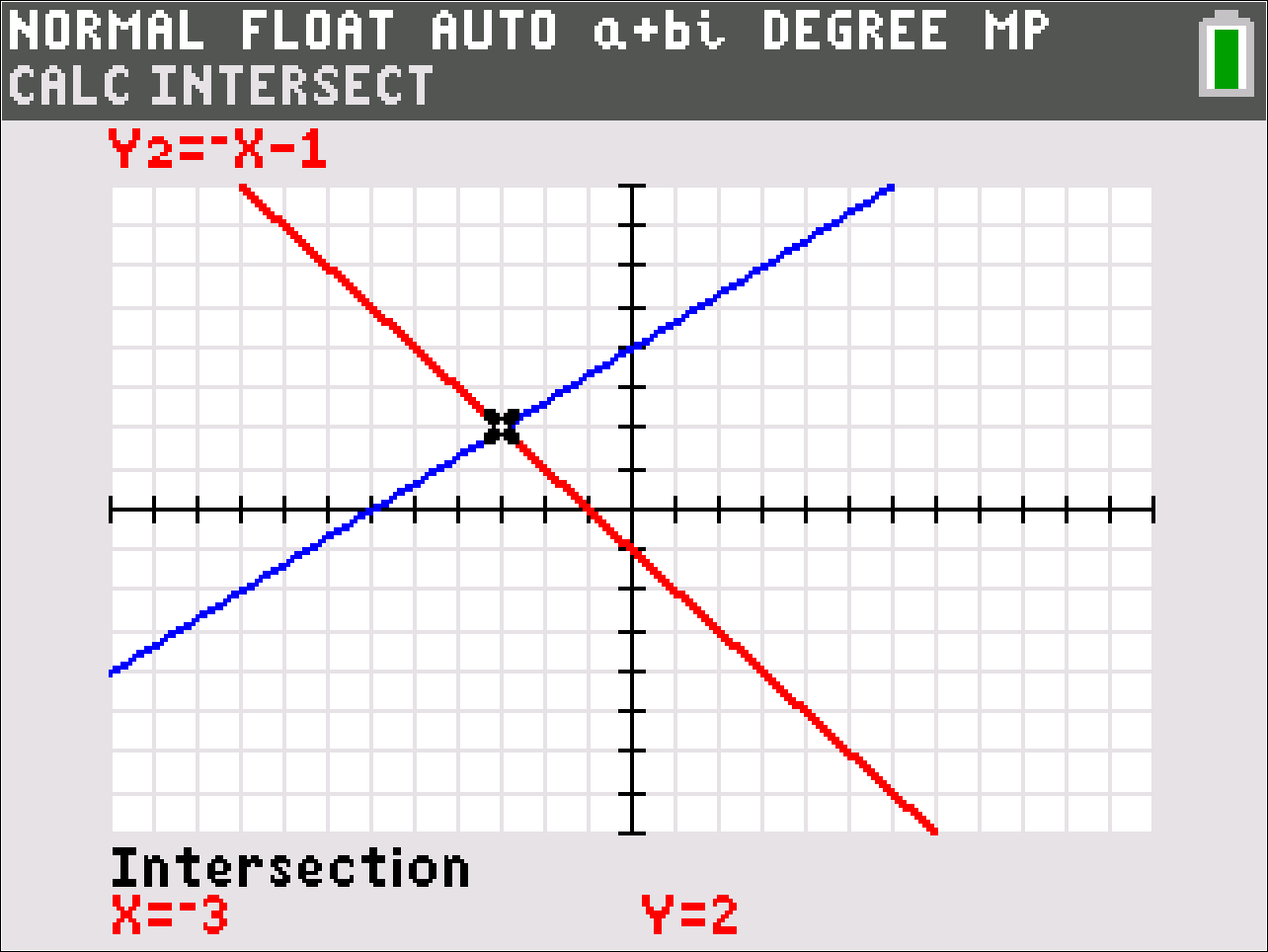10: Solve Systems of Linear Inequalities Graphically

There will also be problems on the ACT that will require you to solve systems of linear inequalities and find the points of intersection in the bounded region. You can solve that problem algebraically and then use the Inequalities (Inequalz) app on the TI-84 Plus to check your solutions. This app even provides the intersection point of the lines. On the ACT, you might see a problem like this:

Solve: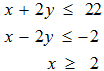Turn on the Inequalities feature by pressing the app key and selecting Inequalz.  Then follow the steps in the video below to check your answer.

*Source: ACT